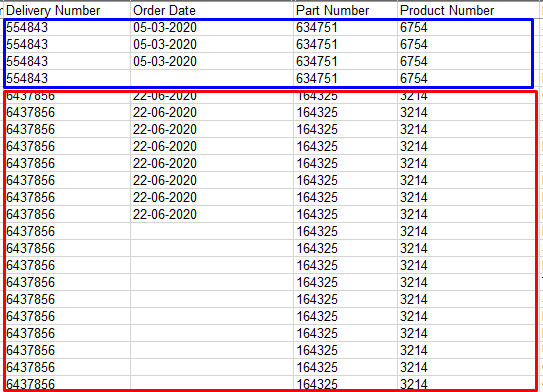# Community

## Field value

May I ask you, can I make the value obtained and found on the first page displayed when exporting for a repeating group in other sheets?
For example, this is a date. It is displayed on the first sheet when unloading values for the entire table. But for the second page, where this date does not exist, the value is empty. Maybe a script? If the value is empty, take from the previous page, I don't know
Thank u community!

•Can you provide a screenshot or the pdf?

•Hey Ekoral. It seems impossible, it didn't work for me with scripts.

•ElliotG Hi mate!
The screenshot shows the recognized and exported values of two documents.
The first document has two sheets. In the second, three. The date is written only on the first sheet of each document. As a result, in the recognized document, it is displayed together with a repeating group only for the first sheet.On the first page only.•That is, I want the value found in the first section to be copied to all the others, if it is empty there.

•May be this script? https://help.abbyy.com/en-us/flexicapture/12/developer/scripting_export_ex But I'm not sure, I couldn't change it correctly

```Section 1
Field1
…
Group1
Field2
…
…
FieldN
Section 2
Field1
…
FieldK

[VBScript]
Dim field1Value, field2Value
' Gets the value of Field1 in Section 1
if Not IsNull( me.Field( "Section 1\Field1" ).Value ) then
field1Value = me.Field( "Section 1\Field1" ).Value
end if
' Gets the value of Field2 in Group1 in Section 1
if Not IsNull( me.FIELD( "Section 1\Group1\Field2" ).Value ) then
field2Value = me.Field( "Section 1\Group1\Field2" ).Value
end if
' Gets the value of Field1 in Section 1 if the section has multiple instances
if Not me.Field( "Section 1" ).Items Is Nothing then
dim curPage
for each curPage in me.Field( "Section 1" ).Items
if Not curPage.Children Is Nothing then
dim curField
for each curField in curPage.Children
if curField.Name = "Field1" And Not IsNull( curField.Value )  then
field1Value = curField.Value
exit for
end if
next
end if
next
end if
[JScript]
// Gets the value of Field1 in Section 1
if( Field("Section 1\\Field1").Value != null ) {
var field1Value = Field("Section 1\\Field1").Value;
}
// Gets the value of Field2 in Group1 in Section 1
if( Field("Section 1\\Group1\\Field2").Value != null ) {
var field2Value = Field("Section 1\\Group1\\Field2").Value;
}
// Gets the value of Field1 in Section 1 if the section has multiple instances
if( Field( "Section 1" ).Items != null ) {
var i, j;
for( i = 0; i < Field( "Section 1" ).Items.Count; i++ ) {
var curPage = Field( "Section 1" ).Items.Item( i );
if( curPage.Children != null ) {
for( j = 0; j < curPage.Children.Count; j++ ) {
var curField = curPage.Children.Item( j );
if( curField.Name == "Field1" && curField.Value != null ) {
var field1Value  = curField.Value;
break;
}
}
}
}
}```